+86
+86

×

+86

×

【小麓讲堂】熔融拉锥型波分复用器简介

1

2

· 熔融拉锥结构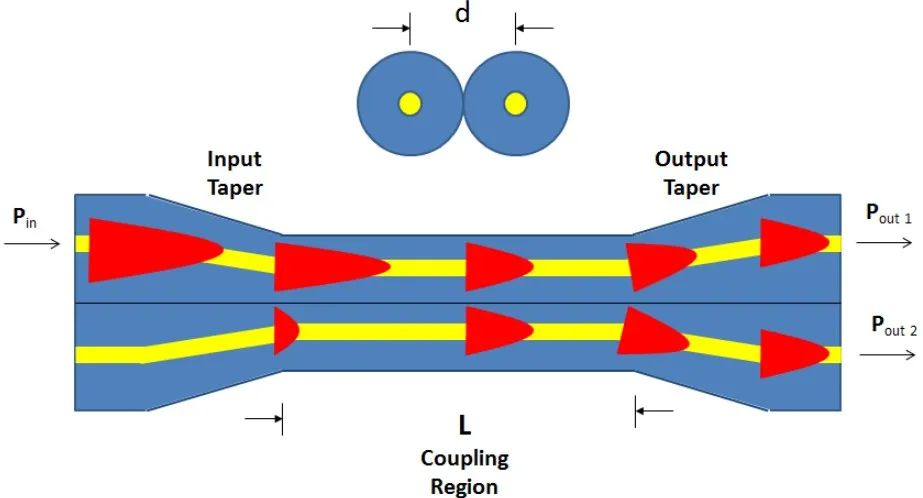· 熔融拉锥工作原理及实例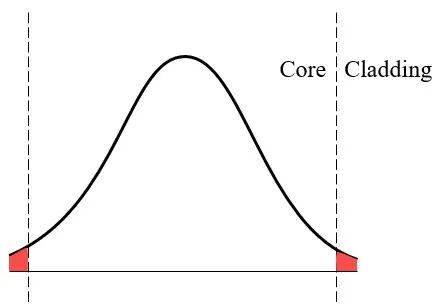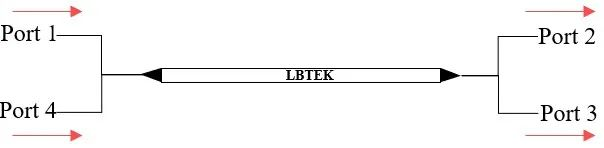3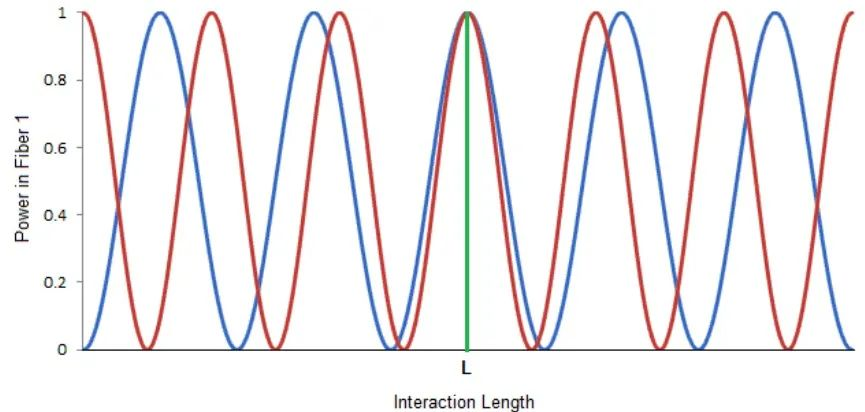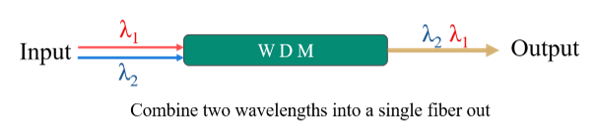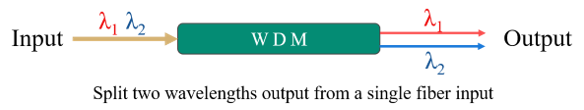4

IL=10lg(Pout/Pin)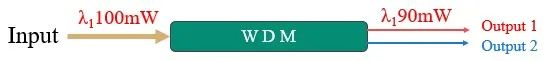IL= 10 × lg (90/100)

= 10 × (-0.046)

= -0.46 dB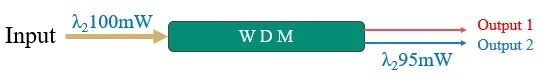IL= 10 × lg (95/100)
= 10 × (-0.022)
= -0.22 dB

Iso=10×lg(Pout/Pin)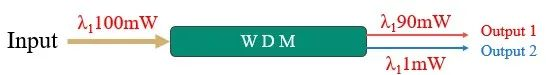Iso = 10 × lg (1/100)

= 10 × (-2)

= -20dB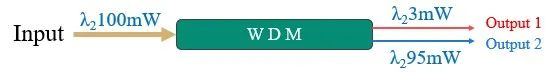Iso = 10 × lg (3/100)

= 10 × (-1.5)

= -15 dB